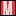# CEF relative discounts and premiums

Relative discounts/premiums are relative to the average discount of a specific closed-end fund (CEF).

•Morningstar

This article presumes an understanding of absolute discounts and premiums. If you need a refresher on absolute discounts, see Closed-end fund discounts and premiums. Here we'll consider relative discounts and relative premiums.

In truth, all discounts and premiums are relative to another number. Absolute discounts/premiums are relative to the net asset value (NAV). Relative discounts/premiums are relative to the average discount of the particular closed-end fund (CEF) being considered. Because absolute discounts and absolute premiums tend to persist, relative discounts and relative premiums matter. Academic studies have shown that current discounts/premiums converge to their average discounts/premiums much more regularly than they converge to their NAVs.

To measure relative discounts, we use a z-score:

z = (current discount - average discount) / standard deviation of the discount

• A negative z-score indicates that the current discount is lower than its average.
• A positive z-score indicates that the current premium is higher than average.

In our opinion, a z-score of less than -2 signals that a fund is relatively inexpensive, and a z-score greater than +2 signals that a fund is relatively expensive.

Example 1

• Current discount = −8%
• Average 1-year discount = −15%
• 1-year standard deviation of discount/premium = 2
• z-score = (-8 - -15) ÷ 2=(-8 + 15) ÷ 2 = 7 ÷ 2 = 3.5

With a z-score of 3.5, this fund would be considered relatively expensive. But this doesn't necessarily mean that the CEF is overvalued.

Example 2

• Current discount = -15%
• Average 1-year discount = -10%
• 1-year standard deviation of discount/premium = 2
• z-score = (-15 - -10) ÷ 2 = (-15 + 10) ÷ 2 = -5 ÷ 2 = -2.5

With a z-score of -2.5, this fund would be considered relatively inexpensive. But this doesn't necessarily mean that the CEF is undervalued. See Accessing the Morningstar report for the z-score for details.

## Why are relative discounts helpful?

For one, they can help you avoid value traps. Let's look at the mythical CEF trading at a 15% discount. According to the oft-cited "CEF wisdom," this would be a good trade because the market is offering investors \$1.00 of assets at the bargain price of \$0.85. (Forget the fact that the \$1.00 worth of assets may fall in value to \$0.85!) Consider this:

• 3-year average absolute discount = -25%
• Current absolute discount = -15%
• Standard deviation over the certain time period = 2
• z-score = (-15 - -25) ÷ 2 = (-15 + 25) ÷ 2 = 10 ÷ 2 = +5

A z-score of +5 indicates that, far from being relatively inexpensive—as CEF wisdom would have it—his CEF is relatively expensive. It could represent a classic value trap.

Z-score can also help investors uncover potentially truly undervalued and overvalued CEFs. If the z-score is greater than +2 or less than -2, more research would be warranted. Using relative discounts/premiums is a bit of an art. The time period analyzed is a large factor in the z-score. The same CEF may look relatively expensive on a 6-week basis and relatively cheap on a 3-year basis. Even though the CEF may look relatively expensive or relatively cheap, it may not be truly overvalued or undervalued.

Consider a CEF that is going to liquidate in one month. Liquidation is a method of making a CEF's share price converge with its NAV. All assets are sold and the remaining capital is distributed to shareholders. At the point of liquidation, the discount will be 0.

• Current discount = -2% (in anticipation of the pending liquidation)
• 1-year average discount = -12%
• 1-year standard deviation = 1.5
• z-score = (-2 - -12) ÷ 1.5 = (-2 + 12) ÷ 1.5 = 10 ÷ 1.5 = +6.7
• This CEF is relatively expensive, but with very good reason: A corporate action has narrowed the discount. If an investor attempted a short sale of this CEF in the market, the likely outcome would be a capital loss.

There could be a fundamental reason behind a high or low z-score. Do not buy or sell a CEF simply because of its z-score. Further analysis as to why the current discount has deviated so far from its historic average is warranted.

## Key takeaways

• Buy-and-hold investors can use z-scores to determine whether the absolute discount/premium is truly signaling that the CEF is under- or overvalued or whether the absolute discount/premium could be a value trap.
• Trading-oriented investors can use z-scores to find candidates for buying or selling short. (In practice, this is the most common use of relative discounts/premiums.)
• Regardless of how they are used, z-scores are no guarantee of future investment gains. All that matters once a CEF is purchased is the subsequent total return. Just as absolute discounts/premiums can converge to NAV with no gain for the shareholder, so too can relative discounts/premiums.
• It is important to understand why a CEF is trading at a current discount/premium that is widely divergent from its historic average. There could be a very good reason, aside from market sentiment.